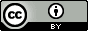## Monday, April 30, 2012

### Computing properties with molecular dynamics

Within statistical mechanics a molecular property $X$ is computed by $$\left<{X}\right>=\sum^{states}_i X_i p_i$$where $X_i$ is the value of $X$ for energy state $i$ and $p_i$ is the probability of being in energy state $i$ with energy $E_i$:$$p_i=\frac{e^{-E_i/kT}}{\sum_i e^{-E_i/kT}}$$Within molecular dynamics (MD) the corresponding property is computed by$$\left<{X}\right>=\frac{1}{M}\sum^M_i X(t_i)$$where $M$ is the number of time-steps and $X(t_i)$ is the value of $X$ at time $t_i$.

For example, the average energy (also called the internal energy $U$) is given by$$\left<{E}\right>=\frac{1}{M}\sum^M_i E(t_i) \text{ where }E(t_i)=\sum^N_k \frac{1}{2}mv^2_k(t_i)+\sum^N_{k,l}V(r_{kl}(t_i))$$where $N$ is the number of particles.  Similarly for the temperature $T$:$$T(t_i)=\frac{1}{k(3N-3)}\sum^N_k \frac{1}{2}mv^2_k(t_i)$$The two most common MD simulations are constant $E$ and constant $T$ simulations: if the step-size is sufficiently small then energy is conserved and $E$ will be constant but $T$ will fluctuate.  Alternative, one can ensure that T is constant (but $E$ fluctuates) by scaling the velocities every so often:$$v_k=\lambda v_k \text{ where }\lambda=\sqrt{\frac{T}{T(t_i)}}$$The heat capacity at constant volume ($C_V$) and pressure ($P$) is computed from, respectively: $$C_V(t_i)=\frac{(E(t_i)-\left<E\right>)^2}{kT^2}$$ and $$P(t_i)=\frac{NkT}{V}-\frac{1}{3V}\sum_{k,l}r_{kl}(t_i)F_{kl}(t_i)$$ where $F_{kl}$ is the force between particle $k$ and $l$.

In principle, the free energy can also be calculated as an average:$$A\propto kT\ln\left(\frac{1}{M}\sum_i^Me^{+E(t_i)/kT}\right)$$but this is not practically feasible because this expression is dominated by high energies, which are rarely sampled.  Instead free energy differences are computed directly from the probabilities.  For example, to compute the free energy difference for the binding of $X$ and $Y$:$$X\bullet Y\leftrightharpoons X+Y$$one computes the amount of time X and Y is bound and unbound and computes $$\Delta G=-RT\ln\left(\frac{\text{time unbound}}{\text{time bound}}\right)$$This work is licensed under a Creative Commons Attribution 3.0 Unported License.